Courses

# Doc: Electric Dipole Class 12 Notes | EduRev

## NEET : Doc: Electric Dipole Class 12 Notes | EduRev

The document Doc: Electric Dipole Class 12 Notes | EduRev is a part of the NEET Course Physics For JEE.
All you need of NEET at this link: NEET

Introduction

• Definition: When two charges of equal magnitude and opposite sign are separated by a very small distance, then the arrangement is called electric dipole.
• Total charge of the dipole is zero but electric field of the dipole is not zero as charges q and -q are separated by some distance and electric field due TO them when added is not zero.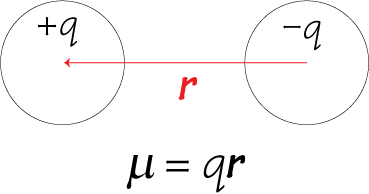We define a quantity called Dipole Moment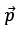for such a system such that: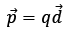where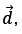conventionally, represents the direction from –q to +q.
Axis of a dipole is the line joining –q to +q

• Midpoint of the axis of the dipole is called the centre of the dipole.
• All the distances in the space are measured from the centre of the dipole.
• Perpendicular bisector of the axis of the dipole is called the equatorial line of the dipole.

Q. Why are we defining a dipole? Why can’t we treat it as simply two charge system? Why are we giving it special treatment?
Ans. Dipole is commonly occurring system in nature. We need to generalize our results in context with the dipole system to avoid repeated single point charge calculations using Coulomb’s Law. After these results we would be able to directly apply simplified results derived here to dipole systems.

Calculation of Electric Field due to an Electric Dipole

1. Field of an electric dipole at points in equatorial plane
We now find the magnitude and direction of electric field due to dipole.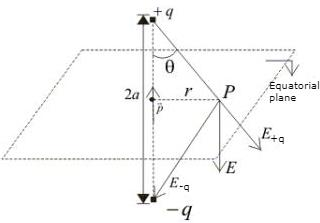• P point in the equatorial plane of the dipole at a distance r from the centre of the dipole. Then electric field due to -q and +q are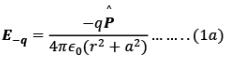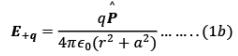and they are equal in magnitude. Note that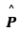is the unit vector along the dipole axis (from -q to + q)

• From figure we can see the direction of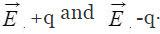Their components normal (perpendicular) to dipole cancel away and components along the dipole add up.
• Dipole moment vector points from negative charge to positive charge so in vector form.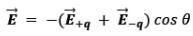Substituting the values of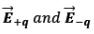calculated above also, by geometry,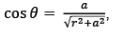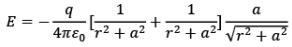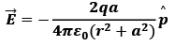Now, very frequently we measure electric field at large distances from the dipole ie. r>>a
Therefore, by approximation,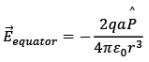We know that, by definition,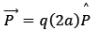Hence,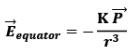Observe, the - sign, it represents that electric field at the equator is in the opposite direction to the dipole moment of the electric dipole i.e. +q to –q.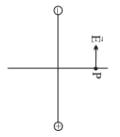2. Field of an electric dipole for points on the axis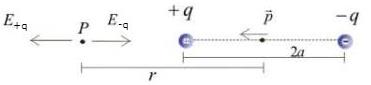• Let P be the point at a distance r from the centre of the dipole on side of charge +q as shown in the figure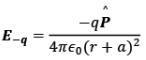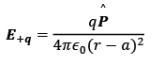Where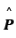is the unit vector along the dipole axis (from -q to + q)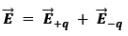Thus,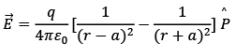or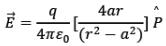for r >> a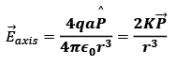As we know that, by definition of dipole moment,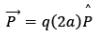• Unit of dipole moment is Coulomb meter (Cm).
• Thus, in a nutshell, in terms of electric dipole moment, electric field due to a dipole at large distances (r >> a)

(i) At point on equatorial plane ( r >> a)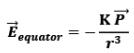(ii) At point on dipole axis (r>>a)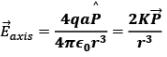Note: Dipole field at large distances falls off as 1/r3.

Now, we can generalize the calculation of electric field at any general point in space due to the dipole using the above results.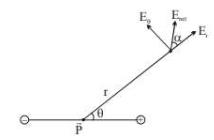Any general point in space, can be located using the polar coordinates r and 𝜃, where the origin can be placed at the center of the dipole, as shown in the above figure.

Now, for any general point P in space located at distance r from centre and inclined at an angle 𝜃 with the axis of the dipole, we can imagine components of the original dipole with dipole moment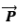such that the P lies on the equator of one component and on the axis of the other component.

Now, Lets express our dipole momentas,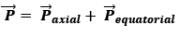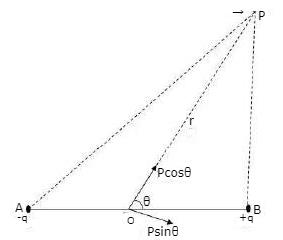Where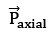is the component of the original dipole moment, such that point P is located on the axis of this dipole, i.e.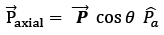Now, at P,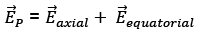We know that,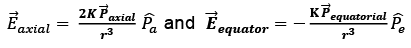Thus,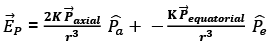Therefore,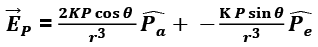One of the component will be along the axial component of electric dipole i.e.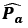and the other component will be along the equatorial component of electric dipole i.e.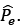ELECTRIC FIELD DUE TO A DIPOLE
The electric field due to a pair of equal and opposite charges at any test point can be calculated using the Coulomb’s law and the superposition principle. Let the test point P be at a distance r from the center of the dipole. The distance between +q and -q is d. We have shown the situation in the diagram below.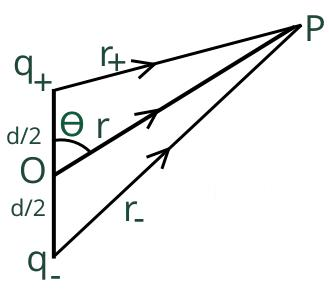I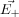an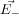be the electric field at point P due to the positive and the negative charges separately then the total electric fiel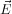at Point P can be calculated by using the superposition principle.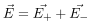Please note that the directions oandare alon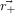and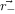respectively. This is the most general form of the electric field due to a dipole. However, we will express this vector in terms of radial and inclination vectors as shown in the diagram below.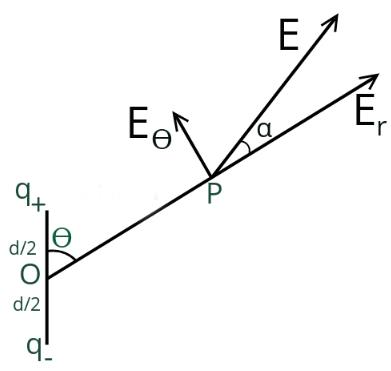In order to calculate the electric field in the polar coordinate, we will use the expression of the electric potential due to an electric dipole which we have calculated earlier.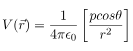Here p is the magnitude of the dipole moment and is given by qd

We can easily derive the electric field due to this dipole by calculating the negative gradient of this electric potential. In polar coordinate electric field will be independent of azimuthal (ϕ) coordinate.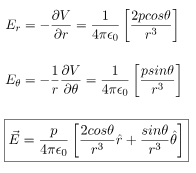The resultant electric field at point P is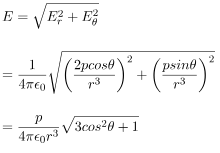As shown in the diagram, the resultant electric field makes an angle \alpha with the radial vector. Then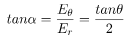ELECTRIC FIELD AT AN AXIAL POINT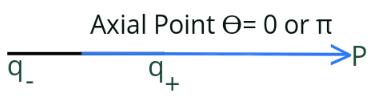In this case, the test point P is on the axis of the dipole. Consequently θ= 0 or π. The electric field at point P is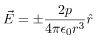SIMPLIFIED DERIVATION
The electric field at point P due to the positive charge is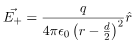Electric field at point P due to negative charge is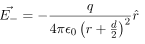Total electric field due to the dipole at axial point P is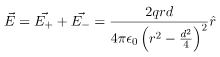At a relatively large distance r>>d/2 and we can approximate the electric field as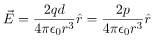ELECTRIC FIELD AT AN EQUATORIAL POINT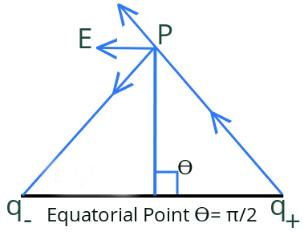In this case, the test point P is on the perpendicular bisector of the dipole. Consequently θ= π/2. The electric field at point P is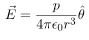SIMPLIFIED DERIVATION
The electric field at point P due to positive charge is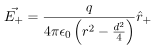Electric field at point P due to negative charge is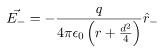Total electric field due to the dipole at equatorial point P is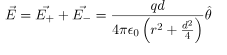At a relatively large distance r>>d/2 and we can approximate the electric field as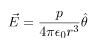CONCEPT OF SOLID ANGLE
Solid angle is a generalisation of the plane angle: In figure we show a plane curve AB. The end points A and B are joined to the point O. We say that the curve AB subtends an angle or a plane angle at O. An angle is formed at O by the two lines OA and OB passing through O. We say that the curve AB subtends an angle or a plane angle at O. An angle is formed at O by the two lines OA and OB passing through O.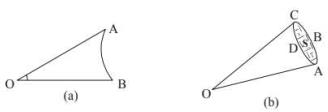To construct a solid angle, we start with a surface S (fig.) and join all the points on the periphery such as A, B, C, D etc., with the given point O. We then say that a solid angle is formed at O and that the surface S has subtended the solid angle. The solid angle is formed by the lines joining the points on the periphery with O. The whole figure looks like a cone. As a typical example, think of the paper containers used by Mungfali Wala.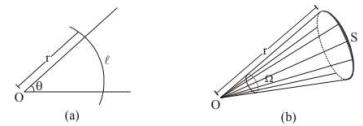How do we measure a solid angle? Let us consider how do we measure a plane angle. See fig. We draw a circle of any radius r with the centre at O and measure the length 𝑙 of the arc intercepted by the angle.
The angle 𝜃 is then defined as 𝜃 = 1/r. In order to measure a solid angle at the point O (fig.), we draw a sphere of any radius r with O as the centre and measure the area S of the part of the sphere intercepted by the cone. The solid angle Ω is then defined as Ω = S/r2

Note: That this definition makes the solid angle a dimensionless quantity. It is independent of the radius of the sphere drawn.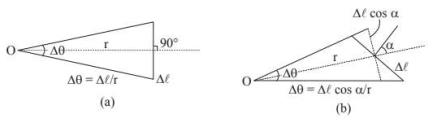Next, consider a plane angle subtended at a point O by a small line segment Δℓ (fig.). Suppose, the line joining O to the middle point of Δℓ is perpendicular to Δℓ.As the segmentis small, we can approximately write.
Δ𝜃 =Δℓ/r
As Δℓ gets smaller, the approximation becomes better. Now suppose, the line joining O to Δℓ is not perpendicular to Δℓ (fig.). Suppose, this line makes an angle 𝛼 with the perpendicular to Δℓ. The angle subtended by Δℓ at O is
Δ𝜃 = Δℓcos 𝛼/r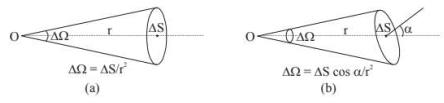Similarly, if a small plane area ΔS (fig.) subtends a solid angle Δℓ at O in such a way that the line joining O to ΔS is normal to ΔS, we can writeΔ Ω = ΔS / r2.
But if the line joining O to ΔS makes an angle 𝛼 with the normal to ΔS (fig.), we should write
ΔΩ = ΔScos 𝛼/r2
A complete circle subtends an angle
𝜃 = ℓ/r = 2𝜋r/r = 2𝜋
at the centre. In fact, any closed curve subtends an angle 2π at an y of the internal points. Similarly, a complete sphere subtends a solid angle,
Ω = S/r2 = 4𝜋𝑟2/r2 = 4𝜋
at the centre. Also, any closed surface subtends a solid angle 4π at any internal point.
How much is the angle subtended by a closed plane curve at an external point?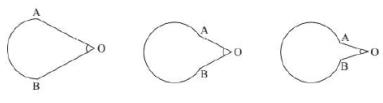See fig. As we gradually close the curve, the angle finally diminishes to zero. A closed curve subtends zero angle at an external point. Similarly, a closed surface subtends zero solid angle at an external point.

APPLICATION OF SOLID ANGLE
Q. Fraction of light emerging from an isotropic point source through a conical region having semi vertex angle 𝛼 and with its apex at the source.
Ans. Let us consider a sphere of radius R with its centre at the source S.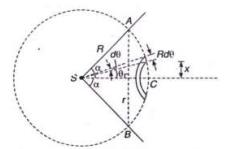Let AB be the section (circular) where the cone ASB intercepts the sphere. If ΔS be the area of the spherical portion ACB (lying within the conical region) then, the solid angle
Ω = ΔS/R2
Let SC be the symmetry axis of the portion of the sphere ACB.
If x be the distance of a thin circular strip then its area
dS = 2𝜋 x Rd𝜃
= 2𝜋 (Rsin 𝜃) Rd𝜃
Total Area,
ΔS = ∫ dS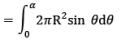= 2𝜋R2 [1 − 𝐜𝐨s 𝜶]
Therefore, area of the curved surface ΔS which subtends an angle 𝛼 at the center,
ΔS = 2𝜋R2 [1 − 𝐜𝐨s 𝜶]
Also, Solid Angle
𝛀 = S/R2 = 2𝜋 [1 − 𝐜𝐨s 𝜶]

This relation between plane angle 𝛼 and solid angle 𝛀 is advised to be remembered.
If Ω steradian be the solid angle for the cone then, the fraction of light passing through the cone will be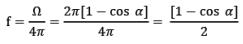Offer running on EduRev: Apply code STAYHOME200 to get INR 200 off on our premium plan EduRev Infinity!

## Physics For JEE

188 videos|491 docs|265 tests

,

,

,

,

,

,

,

,

,

,

,

,

,

,

,

,

,

,

,

,

,

;